Arduino

# How to Create an Arduino-Controlled Calculator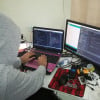November 22, 2018 by Muhammad Aqib
Share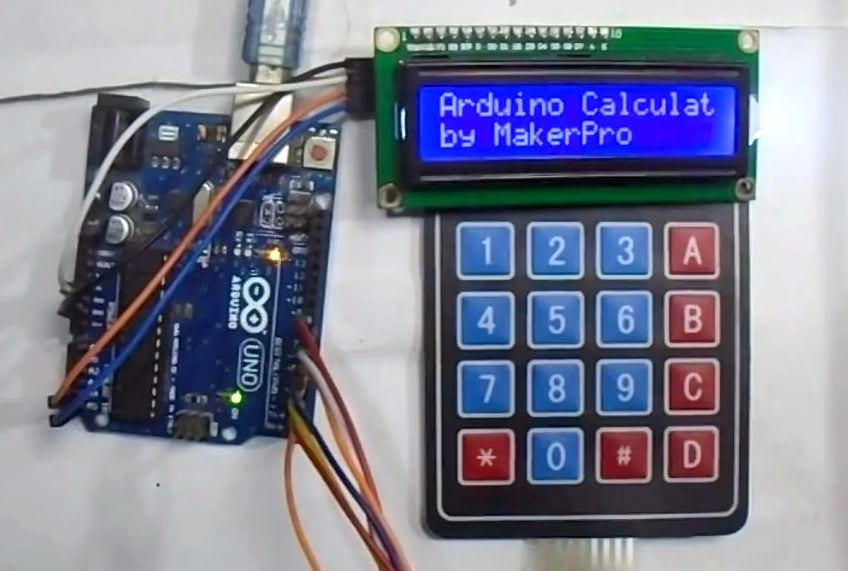Learn how you can create your own calculator using an Ardunio UNO, a 4x4 keypad, and an LCD display!

## Hardware

 1 Arduino UNO 1 4x4 Keypad 1 16x2 I2C LCD Display 1 Jumper Wires

## Software

 1 Arduino IDE

In this post, you are going to learn about how to make an Arduino calculator using 4X4 keypad and Arduino Uno. This calculator will be able to perform simple mathematical operations like addition, subtraction, multiplication, and division. It will also be able to give you the answer in float.

### Circuit Diagram and Explanation

The 4X4 keypad has 8 pins that need to be connected to the Arduino pins from D2 to D9 as follows: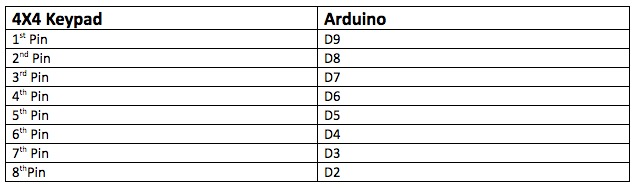Then, connect the LCD with the Arduino as follows: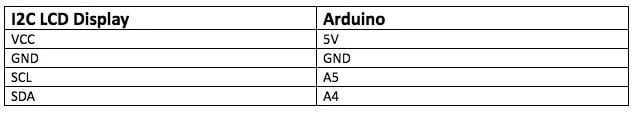The buttons, other than the numerical ones, will perform the following tasks:

• ‘B’ is for subtraction
• ‘C’ is for clear
• ‘D’ is for divide
• ‘*’ is for multiply

The complete circuit diagram is given below.Arduino calculator diagram.

### Code Breakdown and Walkthrough

Let's take a look at the code necessary for this project and what each section of code does. The full code is available at the end of the article.

First, you need to add the libraries for the keypad and I2C LCD display. The LCD display used works with the UNO through the I2C communication, so use the wire library which allows for I2C communication on the Arduino.

Then, follow the pin connections for the 4X4 keypad and the directions for which keypad button performs an operation.

``````#include<Keypad.h>
#include<LiquidCrystal_I2C.h>
#include<Wire.h>

const byte ROWS = 4;
const byte COLS = 4;
char keys[ROWS][COLS] = {
{'1', '2', '3', '+'},
{'4', '5', '6', '-'},
{'7', '8', '9', 'C'},
{'*', '0', '=', '/'}
};

byte rowPins[ROWS] = {9, 8, 7, 6};
byte colPins[COLS] = {5, 4, 3, 2};``````

In the setup function, the display will read “Arduino calculator by MakerPro”.

``````lcd.begin();
lcd.setCursor(0, 0);
lcd.print("Arduino Calculator");
lcd.setCursor(0, 1);
lcd.print("by MakerPro");
delay(1000);
scrollDisplay();
clr();``````

In the loop function, we first get the pressed key and then we need to check whether the key pressed is the numeric one. If it is the numeric one, then it will be stored in the firstNum string.

``````char newKey = myKeypad.getKey();
if (newKey != NO_KEY && (newKey == '1' || newKey == '2' || newKey == '3' || newKey == '4' || newKey == '5' || newKey == '6' || newKey == '7' || newKey == '8' || newKey == '9' || newKey == '0')) {

if (firstNumState == true) {
firstNum = firstNum + newKey;

lcd.print(newKey);
}
else {
secondNum = secondNum + newKey;

lcd.print(newKey);
}``````

If the key pressed is not numeric, check whether it is ‘+’, ‘-‘, ‘/’, ‘*’ (On the keypad, these keys are ‘A’, ‘B’, ‘D’, ‘*’). If it will be from these keys, we will store a value that will be used later on. It will also set the firstNum to false which means we will now get the second number.

Now the further numerical values will be stored in the secondNum string.

``````if (newKey != NO_KEY && (newKey == '+' || newKey == '-' || newKey == '*' || newKey == '/')) {
if (firstNumState == true) {
operatr = newKey;
firstNumState = false;
lcd.setCursor(15, 0);
lcd.print(operatr);
lcd.setCursor(5, 1);
}
}``````

Finally, we set it up so if keys pressed are not from the operation keys, it will check whether it is ‘=’. If it is this key, then it will perform the stored operation on the first and second number and will output the result.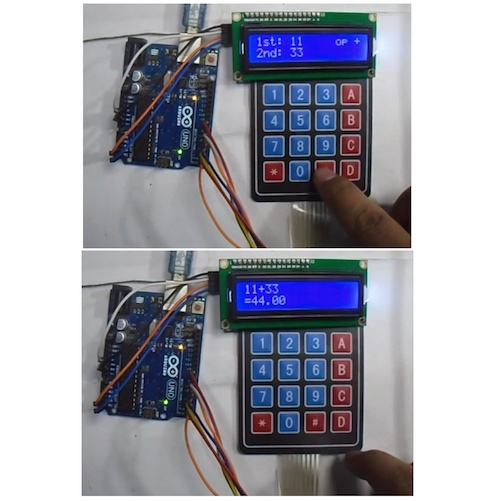After setting up our code, the calculator will be able to perform equations.

``````if (newKey != NO_KEY && newKey == '=') {
if (operatr == '+') {
result = firstNum.toFloat() + secondNum.toFloat();
}

if (operatr == '-') {
result = firstNum.toFloat() - secondNum.toFloat();
}

if (operatr == '*') {
result = firstNum.toFloat() * secondNum.toFloat();
}

if (operatr == '/') {
result = firstNum.toFloat() / secondNum.toFloat();
}
lcd.clear();
lcd.setCursor(0, 0);
lcd.print(firstNum);
lcd.print(operatr);
lcd.print(secondNum);
lcd.setCursor(0, 1);
lcd.print("=");
lcd.print(result);
firstNumState = true;
}

And if the key will be 'C', then it will clear the display screen.
if (newKey != NO_KEY && newKey == 'C') {
clr();
}``````

### Full Calculator Project Code

``````#include<Keypad.h>
#include<LiquidCrystal_I2C.h>
#include<Wire.h>

const byte ROWS = 4;
const byte COLS = 4;
char keys[ROWS][COLS] = {
{'1', '2', '3', '+'},
{'4', '5', '6', '-'},
{'7', '8', '9', 'C'},
{'*', '0', '=', '/'}
};

byte rowPins[ROWS] = {9, 8, 7, 6};
byte colPins[COLS] = {5, 4, 3, 2};

// Created instances
LiquidCrystal_I2C lcd(0x27, 16, 2);

boolean firstNumState = true;
String firstNum = "";
String secondNum = "";
float result = 0.0;
char operatr = ' ';

void setup() {
lcd.begin();
lcd.setCursor(0, 0);
lcd.print("Arduino Calculator");
lcd.setCursor(0, 1);
lcd.print("by MakerPro");
delay(1000);
scrollDisplay();
clr();
}

void loop() {
if (newKey != NO_KEY && (newKey == '1' || newKey == '2' || newKey == '3' || newKey == '4' || newKey == '5' || newKey == '6' || newKey == '7' || newKey == '8' || newKey == '9' || newKey == '0')) {

if (firstNumState == true) {
firstNum = firstNum + newKey;

lcd.print(newKey);
}
else {
secondNum = secondNum + newKey;

lcd.print(newKey);
}
}
if (newKey != NO_KEY && (newKey == '+' || newKey == '-' || newKey == '*' || newKey == '/')) {
if (firstNumState == true) {
operatr = newKey;
firstNumState = false;
lcd.setCursor(15, 0);
lcd.print(operatr);
lcd.setCursor(5, 1);
}
}

if (newKey != NO_KEY && newKey == '=') {
if (operatr == '+') {
result = firstNum.toFloat() + secondNum.toFloat();
}

if (operatr == '-') {
result = firstNum.toFloat() - secondNum.toFloat();
}

if (operatr == '*') {
result = firstNum.toFloat() * secondNum.toFloat();
}

if (operatr == '/') {
result = firstNum.toFloat() / secondNum.toFloat();
}
lcd.clear();
lcd.setCursor(0, 0);
lcd.print(firstNum);
lcd.print(operatr);
lcd.print(secondNum);
lcd.setCursor(0, 1);
lcd.print("=");
lcd.print(result);
firstNumState = true;
}

if (newKey != NO_KEY && newKey == 'C') {
clr();
}
}

void scrollDisplay() {
// scroll 13 positions (string length) to the left
// to move it offscreen left:
for (int positionCounter = 0; positionCounter < 3; positionCounter++) {
// scroll one position left:
lcd.scrollDisplayLeft();
// wait a bit:
delay(300);
}
delay(1000);

// scroll 29 positions (string length + display length) to the right
// to move it offscreen right:
for (int positionCounter = 0; positionCounter < 3; positionCounter++) {
// scroll one position right:
lcd.scrollDisplayRight();
// wait a bit:
delay(300);
}
delay(2000);
}

void clr() {
lcd.clear();
lcd.setCursor(0, 0);
lcd.print("1st: ");
lcd.setCursor(12, 0);
lcd.print("op ");
lcd.setCursor(0, 1);
lcd.print("2nd: ");
lcd.setCursor(5, 0);
firstNum = "";
secondNum = "";
result = 0;
operatr = ' ';
}``````